MIDAS: The "golden touch" for
Microwave Inductors Design
Automation on Silicon

 MIDAS can cover the range of inductances from about 0.1 to 5nH according to the following approximated formula :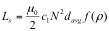(1) where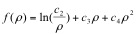(2)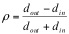(3)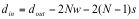(4)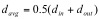(5) Where N is the number of turns, w is the track width, s is the spacing between adjacent tracks dout, din and davg refer to the outer, inner and average diameter of the spiral to be drawn. The coefficients c1, c2, c3 and c4 given in Table 1 allow the formula to be adapted to both octagonal and square spiral inductors.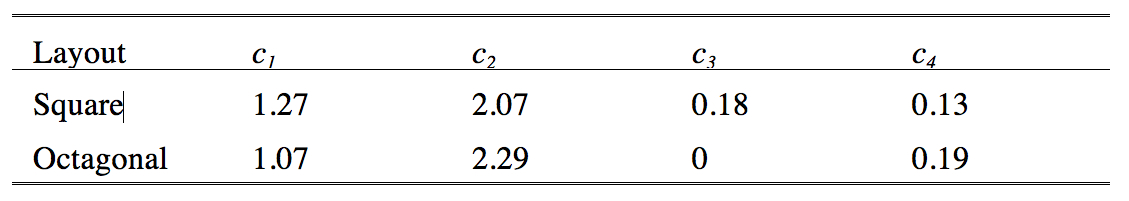Table 1. Values of the coefficients.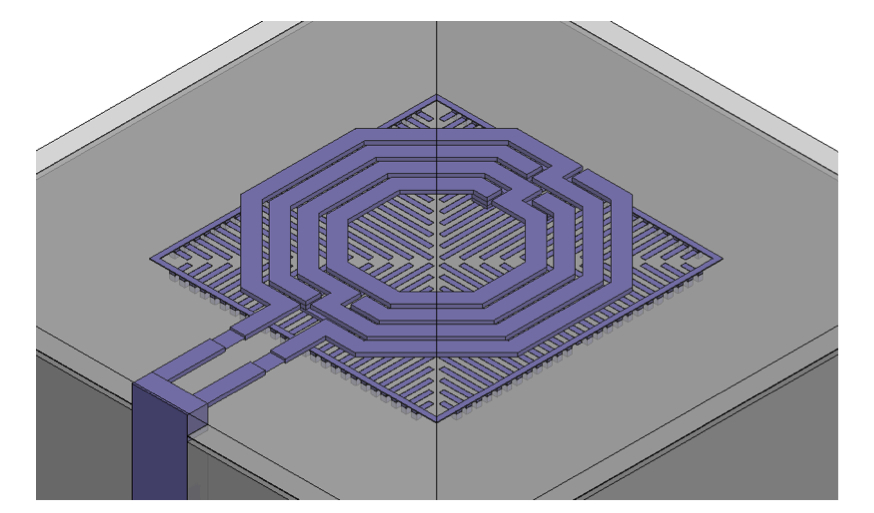Figure 2. Example of an octagonal spiral inductor with PGS created by the EM Structure Simulator. HOME||1 2 3 4